# [新闻] 深入Java源码剖析之字符串常量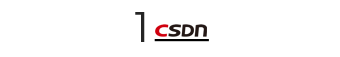Java中是如何实现字符串的

``````/**
* Initializes a newly created {@code String} object so that it represents
* the same sequence of characters as the argument; in other words, the
* newly created string is a copy of the argument string. Unless an
* explicit copy of {@code original} is needed, use of this constructor is
* unnecessary since Strings are immutable.
*
* @param  original
*         A {@code String}
*/
public String(String original) {
this.value = original.value;
this.hash = original.hash;
}``````

``````/** The value is used for character storage. */
private final char value[];``````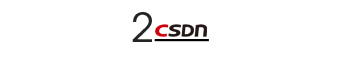``````String s1 = "abc";
String s2 = "abc";``````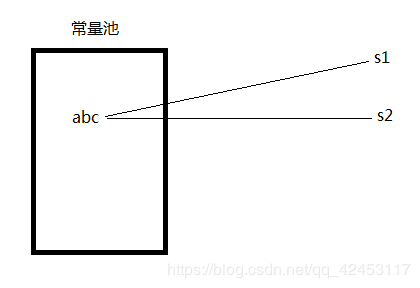``````String s1 = "abc";
String s2 = "abc";
System.out.println(s1 == s2);
``````

``````String s3 = new String("abc");
String s4 = new String("abc");``````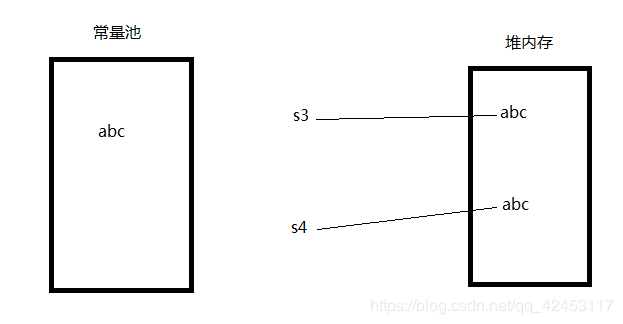``````String s3 = new String("abc");
String s4 = new String("abc");
System.out.println(s3 == s4);
``````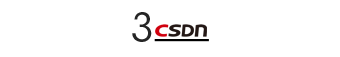``````String s3 = new String("abc");
String s4 = new String("abc");
System.out.println(s3.intern() == s4.intern());``````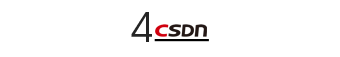JVM内存结构

JVM分为堆、栈、方法区，栈又分为本地方法栈和Java栈。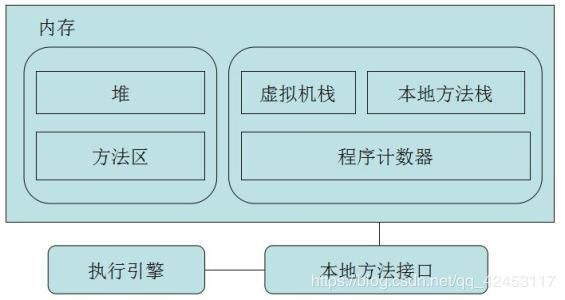``````String s1 = new String("hello") + new String("world");
String s2 = "helloworld";
System.out.println(s1 == s2);``````

``````String s1 = new String("hello") + new String("world");
System.out.println(s1.intern() == s1);``````

``````String s1 = new String("hello") + new String("world");
System.out.println(s1.intern() == s1);
String s2 = new String("hello") + new String("world");
System.out.println(s2.intern() == s2);``````

``````true
false``````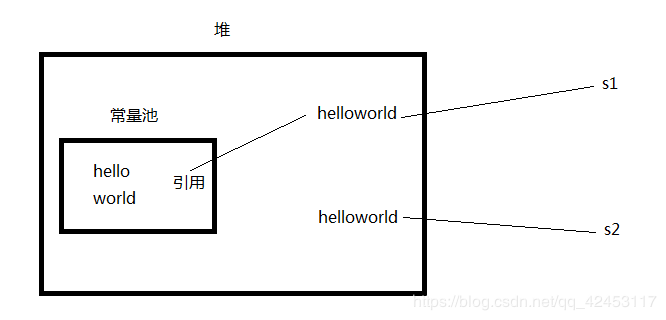``````String s1 = new String("hello") + new String("world");
System.out.println(s1.intern() == s1);
String s2 = new String("hello") + new String("world");
System.out.println(s2.intern() == s1);``````

``````true
true``````

``````List list = new ArrayList();
String str = "boom";
for(int i = 0;i < Integer.MAX_VALUE;i++) {
String temp = str + i;
str = temp;
}``````

``````Exception in thread "main" java.lang.OutOfMemoryError: Java heap space
at java.util.Arrays.copyOfRange(Arrays.java:2694)
at java.lang.String.<init>(String.java:203)
at java.lang.StringBuilder.toString(StringBuilder.java:405)
at com.itcast.test2.StringTest.main(StringTest.java:25)``````

``````Exception in thread "main" java.lang.OutOfMemoryError: PermGen space
at java.util.Arrays.copyOfRange(Arrays.java:2694)
at java.lang.String.<init>(String.java:203)
at java.lang.StringBuilder.toString(StringBuilder.java:405)
at com.itcast.test2.StringTest.main(StringTest.java:25)``````

PermGen space其实就是方法区， 那么其实在JVM中的堆，一般分为三大部分：新生代、老年代、永久代：这个PermGen space就是永久代，也就是方法区，叫法不同而已。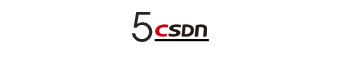``````String s1 = "hello" + "world";
String s2 = "helloworld";
System.out.println(s1 == s2);

String temp = "hello";
String s3 = temp + "world";
String s4 = "helloworld";
System.out.println(s3 == s4);``````

``````true
false``````

``````String s1 = "helloworld";
String s2 = "helloworld";
System.out.println(s1 == s2);

String temp = "hello";
String s3 = String.valueOf(temp) + "world";
String s4 = "helloworld";
System.out.println(s3 == s4);``````

https://blog.csdn.net/qq_42453117/article/details/97128658